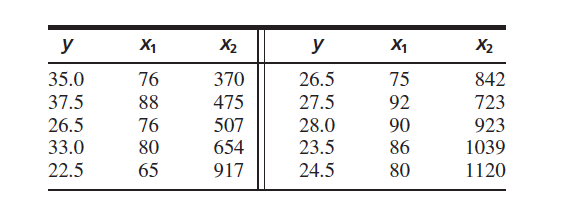×
Get Full Access to Statistics For Engineers And Scientists - 4 Edition - Chapter 8.3 - Problem 15e
Get Full Access to Statistics For Engineers And Scientists - 4 Edition - Chapter 8.3 - Problem 15e

×

# Solved: The article “Vehicle-Arrival Characteristics atISBN: 9780073401331 38

## Solution for problem 15E Chapter 8.3

Statistics for Engineers and Scientists | 4th Edition

• Textbook Solutions
• 2901 Step-by-step solutions solved by professors and subject experts
• Get 24/7 help from StudySoup virtual teaching assistantsStatistics for Engineers and Scientists | 4th Edition

4 5 1 389 Reviews
26
2
Problem 15E

The article “Vehicle-Arrival Characteristics at Urban Uncontrolled Intersections” (V. Rengaraju and V. Rao, Journal of Transportation Engineering, 1995:317–323) presents data on traffic characteristics at 10 intersections in Madras, India. The following table provides data on road width in m $$(x_1)$$, traffic volume in vehicles per lane per hour $$(x_2)$$, and median speed in km/h $$(x_3)$$.a. Fit the model $$y=\beta_{0}+\beta_{1} x_{1}+\beta_{2} x_{2}+\varepsilon$$. Find the P-values for testing that the coefficients are equal to 0.

b. Fit the model $$y=\beta_{0}+\beta_{1} x_{1}+\varepsilon$$. Find the P-values for testing that the coefficients are equal to 0.

c. Fit the model $$y=\beta_{0}+\beta_{1} x_{2}+\varepsilon$$. Find the P-values for testing that the coefficients are equal to 0.

d. Which of the models (a) through (c) do you think is best? Why?

Equation Transcription:Text Transcription:

(x_1)

(x_2)

(x_3)

x_1

x_2

x_1

x_2

y=beta_0+beta_1x_1+beta_2x_2+varepsilon

y=beta_0+beta_1x_1+varepsilon

y=beta_0+beta_1x_2+varepsilon

Step-by-Step Solution:
Step 1 of 3

Rachel Booth 90­944­9011 Eager, Keen, Genuine, and Ready Mississippi State is eager. When I walked on Mississippi State’s campus the faculty and students introduced me to their quiet, steady, formidable drive to be more, expand further, lift each other higher, present and earn more opportunities. While pleased with State’s success, the community wanted more – more recognition, more awards, more skill, more, better, stronger, fiercer, more. I am keen. I relish the idea of sitting beside potential Rhodes Scholars, Truman Scholars, and I know that MSU is going to invest its time, resources, and creativity into me that I may be able to compete for those things as well. They will try to get me into a semester at Oxford, acceptance into Stanford, and the experience and knowledge to thrive at those places. MSU is genuine. I noticed it when an alum heard I was searching for a college and visited my work to give me a package of MSU information and his own campus guide. I felt it when an administrator took me on a personalized tour of campus on his day off – the week before his thesis was due. I loved it when a professor told a girl that MSU didn’t have exactly the major she wanted but he knew of another school that did and he hoped that she was accepted there. MSU is my perfect school. As their student, I am not a youngster trying to find my place in the world ­ lost and scared; I am a bulldog winning my victories because of the care and efforts of my State fa

Step 2 of 3

Step 3 of 3

##### ISBN: 9780073401331

This full solution covers the following key subjects: characteristics, coefficients, fit, Testing, values. This expansive textbook survival guide covers 153 chapters, and 2440 solutions. The answer to “?The article “Vehicle-Arrival Characteristics at Urban Uncontrolled Intersections” (V. Rengaraju and V. Rao, Journal of Transportation Engineering, 1995:317–323) presents data on traffic characteristics at 10 intersections in Madras, India. The following table provides data on road width in m $$(x_1)$$, traffic volume in vehicles per lane per hour $$(x_2)$$, and median speed in km/h $$(x_3)$$. a. Fit the model $$y=\beta_{0}+\beta_{1} x_{1}+\beta_{2} x_{2}+\varepsilon$$. Find the P-values for testing that the coefficients are equal to 0.b. Fit the model $$y=\beta_{0}+\beta_{1} x_{1}+\varepsilon$$. Find the P-values for testing that the coefficients are equal to 0.c. Fit the model $$y=\beta_{0}+\beta_{1} x_{2}+\varepsilon$$. Find the P-values for testing that the coefficients are equal to 0.d. Which of the models (a) through (c) do you think is best? Why?________________Equation Transcription:Text Transcription:(x_1)(x_2)(x_3)x_1x_2x_1x_2y=beta_0+beta_1x_1+beta_2x_2+varepsilony=beta_0+beta_1x_1+varepsilony=beta_0+beta_1x_2+varepsilon” is broken down into a number of easy to follow steps, and 123 words. This textbook survival guide was created for the textbook: Statistics for Engineers and Scientists , edition: 4. Statistics for Engineers and Scientists was written by and is associated to the ISBN: 9780073401331. The full step-by-step solution to problem: 15E from chapter: 8.3 was answered by , our top Statistics solution expert on 06/28/17, 11:15AM. Since the solution to 15E from 8.3 chapter was answered, more than 409 students have viewed the full step-by-step answer.

Unlock Textbook Solution